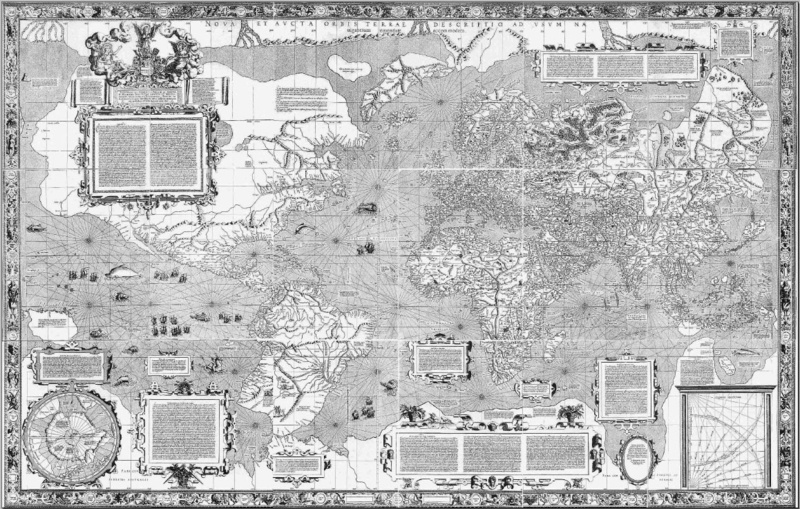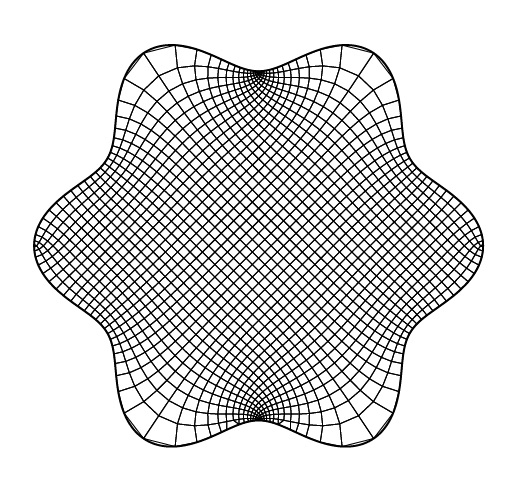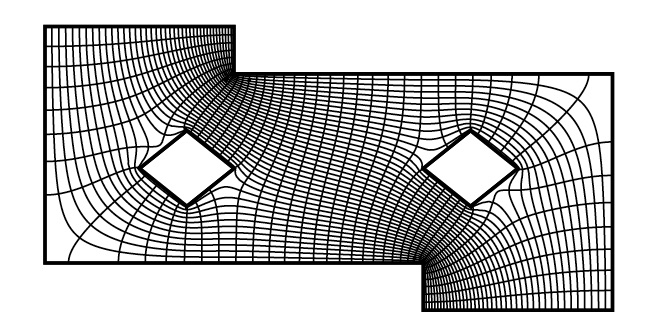# Conformal mappings, numerical analysis and mathematical modeling

Antti Rasila

Department of Mathematics and Systems Analysis

Aalto University

Conformal mappings are a class of functions studied in the classical complex analysis, which are defined by the property that they preserve angles between smooth curves. The basic properties of the complex multiplication along with the chain rule give another important characterization of conformal mappings: a mapping is conformal at the point if and only if it has a non-zero complex derivative at this point. In complex analysis, it is usually assumed that a conformal mapping is a homeomorphism onto its image set. A necessary and sufficient condition for this is that it is univalent (one-to-one).

Conformal mappings can be also studied on Riemannian surfaces and, indeed, a very classical example of conformal mapping between surfaces is Mercator’s conformal map projection, which was first presented in 1569. A rigorous mathematical construction of Mercator’s projection was published by Edward Wright in 1599. Because this was well before the differential and integral calculus, the construction was based solely on the geometric properties of the mapping.Figure 1: Mercator’s map (1569)

Obviously, conformal mappings are particularly useful in cartography, because conformality of the projection means that the angles measured from the terrain correspond to those on the map, i.e., the local geometry of the terrain is preserved by the projection. This is an example of conformal invariance, which is a property that frequently occurs in the nature, and leads to many classical applications of conformal mappings in, e.g., electrostatics, thermodynamics and fluid mechanics.

On the other hand, obtaining analytic expressions of conformal mappings between given planar domains is, except certain special cases, difficult and often impossible. For this reason, developing computational methods for numerical approximation of conformal mappings has recently been an active field of investigation. One popular method is based on the Schwarz-Christoffel formula, which gives a way to compute a mapping from a polygonal domain onto a disk . A MATLAB implementation of this method is available from .

Another approach, introduced in , makes use of the so-called generalized quadrilaterals, which are Jordan domains with four positively oriented points marked on their boundary. These concepts arise from the theory of quasiconformal mappings. By using certain classical results in this theory, one may observe that the mapping of the form

f(z)=u(z)+dv(z)

is a conformal mapping of the Jordan domain Ω onto a rectangle Rd=(0,1) x (0,h), where u,v are the (unique) harmonic solutions of the mixed boundary value problems (see Figure 2 and Figure 3) related to quadrilaterals Ω; z1, z2, z3, z4, and Ω; z2, z3, z4, z1,  respectively, and d is the conformal modulus of the former quadrilateral. The potential functions u,v can be approximated by using any standard PDE solver (see e.g. ), and the modulus d can be obtained from u. The choice of the points z1,…, z4 is arbitrary, but it affects the mapping as these points are mapped on the corners of the rectangle. See also [5,6,7].Figure 2. Dirichlet-Neumann (mixed boundary value) problemThe main advantages of this method are that it works for domains of arbitrary shape and it is easy to implement by using standard PDE solvers. For example, a simple implementation using the Mathematica built in solver is presented in . The algorithm also works on doubly connected domains, and a generalization for multiply connected domains has been studied in .Figure 4. Mapping of a non-polygonal domain onto a rectangleFigure 5. Mapping of a domain with a zero angleFigure 6. Mapping of a doubly connected domain onto an annulusFigure 7. Multiply connected domain 

An example of a new engineering application related to numerical conformal mappings is in optimization problem of a cellular phone network, where users are grouped into different cells and served by different base stations that provide wireless access to the network. Service demand is usually very heterogeneous, non-uniformly distributed, and dynamic, which makes planning, analysis, and optimization of the network difficult. In order to help with these tasks, one may perform the optimizations in a framework based on canonical (e.g. rectangular) domains and spatial mappings (e.g., conformal and related classes of mapping). See .Figure 8. Spatial mapping of the network topology 

References

 T.A. Driscoll, L.N. Trefethen, Schwarz-Christoffel Mapping, Cambridge Monographs on Applied and Computational Mathematics, 8. Cambridge University Press, 2002.

 T.A. Driscoll, Schwarz-Christoffel toolbox for MATLAB, http://www.math.udel.edu/~driscoll/SC/.

 H. Hakula, T. Quach, A. Rasila: Conjugate Function Method for Numerical Conformal Mappings. J. Comput. Appl. Math. 237 (1) (2013), 340-353.

 H. Hakula, A. Rasila, M. Vuorinen: On moduli of rings and quadrilaterals: algorithms and experiments. SIAM J. Sci. Comput. 33 (1) (2011), 279-302.

 L.V. Ahlfors: Conformal invariants: topics in geometric function theory, McGraw-Hill Book Co., 1973.

 O. Lehto, K.I. Virtanen, Quasiconformal mappings in the plane, Springer, Berlin, 1973.

 N. Papamichael, N. Stylianopoulos: Numerical Conformal Mapping: Domain Decomposition and the Mapping of Quadrilaterals. Singapore: World Scientific, 2010.

 J. Lehtonen: Collocation method for solving stochastic elasticity problems with an uncertain domain. Master’s Thesis. Department of Mathematics and Systems Analysis. Aalto University School of Science, 2015. https://aaltodoc.aalto.fi/handle/123456789 /15338.

 H. Hakula, T. Quach, A. Rasila: Conjugate Function Method and Conformal Mappings in Multiply Connected Domains. Preprint. arXiv:1502.02047

 D. González G., H. Hakula, A. Rasila, J. Hämäläinen: Spatial Mappings for Planning and Optimization of Cellular Networks. To appear in IEEE/ACM Transactions on Networking. arXiv:1611.01294